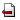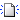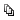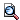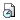• 追加された行はこの色です。
• 削除された行はこの色です。
```第 201 回 Q-NA セミナー
#include(QNA322,notitle)

In this talk we are concerned about singular boundary value problems
arising in hydrodynamics and cosmology. In the case of spherical simmetry,
the orginal partial differential equation may be reduced to a second
order ordinary differential equation (ODE). This is the case, for example,
of the formation of bubbles or droplets in a mixture gas-liquid.
We are interested on solutions of the resulting ODE which are strictly
increasing on the positive semi-axis and have finite limits at 0 and
∞ (bubble-type solutions). Necessary and sufficient conditions for
the existence of such solutions are obtained in the form of a restriction on
the equation coefficients. The asymptotic behavior of certain solutions of
this equation is analysed near the two singularities (when r→0+ and r→∞),
where the considered boundary conditions define one-parameter families of
solutions. Based on the analytic study, an efficient numerical method is
proposed to compute approximately the needed solutions of the above problem.
Some results of the numerical experiments are displayed and their physical
interpretation is discussed.

```

ご不明な点がございましたら、事務局: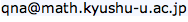までお問い合わせ下さい。